## KCSE 2019 Mathematics Paper 1 Questions With Marking Scheme

SECTION I (50 marks)

Answer all the questions in this section

1. Without using mathematical tables or calaculator, evaluate: (3 marks)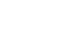2. Express 1728 and 2025 in terms of their prime factors and hence evaluate: (4 marks)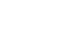3. Juma left his home at 8.30 a.m. he drove a distance of 140km and arrived at his aunt's home at 10.15 a.m. Determine the average speed inkm/h for juma's journey. (3 marks)
4. Expand and simplify. (2 marks)
4(q + 6) + 7(q − 3)
5. In the trapezium PQRS shown below, PQ = 8 cm and SR = 6 cm.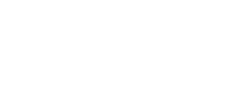If the area of the trapezium is 28 cm2, find the perpendicular distance between PQ and SR. (2 marks)
6. Given that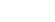, find the value of n. (3 marks)
7. Three villages A, B and C are such that B is 53 km on abearing of 29.5o from A and C is 75 km east of B
1. Using a sclae of 1 cm to represent 10 km, draw a diagram to show the relative positions of villages A, B and C (2 marks)
2. Determine the distance, in km, of C from A
8. A retailer bough a bag of tea leaves. If the retailer were to repack the tea leaves into smaller packets of either 40g, 250g or 350g, determine the least mass, in grams, of the tea leaves in the bag. (3 marks)
9. Given that sin 2x = cos(3x − 10o), find tan x, correct to 4 significant figures. (3 marks)
10. A tourist converted 5820 US dollars into Kenyan shillings at the rate of Ksh 102.10 per dollar. While in Kenya, he spent Ksh 450 000 and converted the balance at the rate of Ksh 103. 00 per dollar. Calculate the amount of money, to the nearest dollar, that remained. (3 marks)
11. Given that b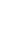, c=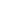and a = 3c − 2b, find the magnitude of a, correct to 2 decimal places. (4 marks)
12. Using a ruler and pair of compasses only, construct a rhombus PQRS such that PQ = 6 cm and ∠SPQ = 75o. Measure the length of PR. (4 marks)
13. Solve the inequality 2x − 1 ≤ 3x + 4 < 7 − x. (3 marks)
14. Given that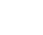,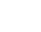and that AB is a singular matrix, find the value of x. (3 marks)
15. A trader bought two types of bulbs A and B at Ksh 60 and Ksh 56 respectively. She bought a total of 50 bulbs of both types at a total of Ksh 2872. Determine the number of type A bulbs that she bought. (3 marks)
16. A bus plies between two towns P and R via town Q daily. On each day it departs from P at 8.15 a.m and stops for 40 minutes at Q before proceeding to R.
On a certain day, the bus took 5 hours 40 minutes to travel from P to Q and 3 hours 15 minutes to travel from Q to R. Find, in 24 hour clock system, the time the bus arrived at R. (3 marks)

SECTION II

Answer any five questions from this section

1. A rectangular water tank measures 2.4 m long, 2m wide and1.5 m high. The tank contained some water up to a height of 0.45 m.
1. Calculate the amount of water, in litres, needed to fill up the tank. (3 marks)
2. An inlet pipe was opened and water let to flow into the tank at a rate of 10 itres per minute. After an hour, a drain pipe was opened water alowed to flow out of the tank at a rate of 4 litres per minute.
Calculate:
1. the height of water in the tank after 3 hours.(4 marks)
2. the total time taken to fill up the tank. (3 marks)
2.
1. A line L1 passes through the points (3,3) and (5,7), Find the equation of L1 in the form y = mx + c, where m and C are constants. (3 marks)
2. Another line L2 is perpendicular to L1 and passes through (−2, 3). Find:
1. the equation of L(3 marks)
2. the x-intercept of L2 (1 mark)
3. Determine the point of intersection of L1 and L2 (3 marks)
3.  A triangle ABC with vertices A (−2, 2), B(1,4) and C(−1,4) is mapped onto a triangle A'B'C' by a reflcetion in the line y = x + 1
1. On the grid provided draw
1. triangle ABC; ( 1 mark)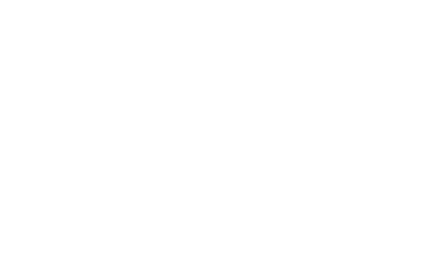2. the line y = x + 1 (2 marks)
3. triangle A'B'C'
2. Triangle A''B''C'' is the image of triangle A'B'C' under a negative quarter turn about (0,0).
On the same grid, draw triangle A"B"C''(3 marks)
3. State the type of congruence between triangles
1. ABC and A'B'C' (1 mark)
2. A'B'C' and A"B"C" (1 mark)
4. The figure below is a right pyramid VEFGH with a square base of 8 cm and a slant edge of 20cm. Points A, B, C and D lie on the slant edges of the pyramid such that VA = VB =VC = VD= 10 cm and plane ABCD is parallel to the base EFGH.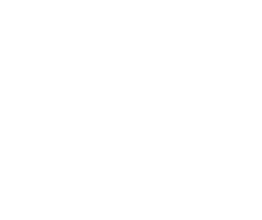1. FInd the length of AB (2 marks)
2. Calculate correct to 2 decimal places:
1. the length of AC (2 marks)
2. the perpendicular height of the pyramid VABCD (2 marks)
3. The pyramid VABCD was cut off. Find the volume of the frustrum ABDCDEFGH correct to 2 decima places. (4 marks)
5. The heights of 40 athletes in acounty athletics competition were as shown in the table below:
 Height, cm Frequency 150 - 159 2 160 - 169 8 170 - 179 10 180 - 189 x 190 - 199 6 200 - 209 2
1. Find the value of x (1 mark)
2. State the modal class (1 mark)
3. Calculate
1. the mean height of the athletes (4 marks)
2. the median height, correct to 1 decimal place, of the athletes. (4 marks)
6. The figure below represents a traingular flower garden ABC in which Ab = 4 m, BC = 5 m and ∠ BCA = 30o. Point D lies on AC such that BD = 4m and ∠ BDC is obtuse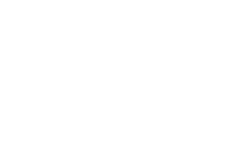Find correct to 2 decimal paces:
1. ∠ BDC (3 marks)
2. the length of AD (3 marks)
3. the length of DC (2 marks)
4. the area of the flower garden ABC (2 marks)
7. The shaded region on the graph below shows a piece of land ABCD earmarked for building a sub-county hospital.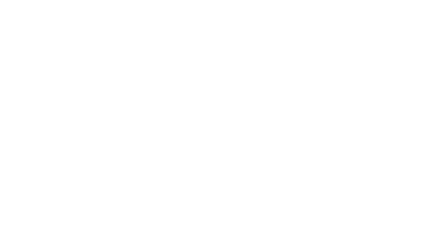1. Write down the ordinates of curves AB and DC for x = 0, 200, 400, 600, 800, 1000 and 1200 (2 marks)
2. USe trapezium rule, with 6 strips to estimate the area of the piece of land ABCD in hectares. (4 marks)
3. Use mid-ordinate rule with 3 strips to estimate the area of the piece of land, in hectares (4 marks)
8. The equation of a curve is y = x3 + x2 − x − 1
1. Determine:
1. the stationary points of the curve; (3 marks)
2. the nature of the stationary points in (a)(i) above. (2 marks)
2. Determine
1. the equation of the tangent to the curve at x = 1; (3 marks)
2. the equation of the norma to the curve at x = 1. (2 marks)

## MARKING SCHEME

1.       5.4         =                 5.4x104
0.025 x 3.6      0.025 x 3.6 x 104

54 x 1000
25 x 36

6000
100
= 60
2. 1728=2x 33
2025=34 x 52

³√1728³√2⁶ x 3³  = 2² x 3
√2025      √34 x 52      32 x 5

4/15 = 0.2666666
3. Time taken =
10.15
8.30
1.45
1 hr 45 mins = 1.75hrs = 1¾
Speed =   140
1.75
= 80 km/h

4. 4(q+6)+7(q -3) = 4q + 24 + 7q -21
= 11q +3

5. Area of trapezium
½(8+6)h = 28
7h = 28
h = 4cm

6. ³√9⁴ =3n
(3²)⁴/³ = 3n
3⁸/³= 3n
n= 8/3=2 ²/₃
7.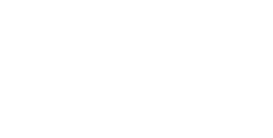8. 40=2 x 2 x 2 x 5
250 = 2 x 5 x 5 x 5
350 = 2 x 5 x 5 x 7
LCM = 2 x 2 x 2 x 5 x 5 x  5 x 7
= 7000g

9. sin 2x = cos(3x-10)
2x+(3x – 10) = 90
5x=100
x=20°
tan 20°=0.3640
10. \$5820 = Ksh (5820 x 102.10)
= Ksh 594222
Balance in \$
594222-450000
103.0
=144222
103
= 1400 US Dollars
11.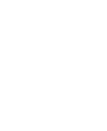|a| = √52+(-2)2
MI
= 5.39
12.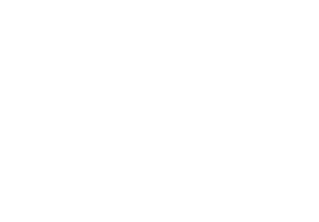PR = (9.5 ± 0.1)cm
13. 2r - 1 < 3x+4
-5 < x
3x+4 < 7- x
4x < 3
x< 3/4
-5 < x < 3/4

14.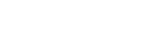|2x+6 11 | = 0
|4x+8 16 |

16(2x+6)-11(4x+8) = 0
32x+96-44x-88 = 0
32x-44x = 88-96
-12x = -8
x = 2/3 = 0.666666

15. A+B= 50
60A +56B = 2872
60A +56(50-A)=2872
4A = 2872-2800
4A = 72
A = 18
16. Time taken =
5 hours 40 min
3 hours 15 min
40 min
9 hours 35min
Arrival time = 08.15 + 9 hrs 35 min
= 1750 hours

17.
1. Volume of water required
= 2.4 x 2 x (1.5 -0.45)m³
=2.4 x 2 x 1.05m³
Amount of water in litres
= 2.4 x 2 x 1.05 x 1000 litres
MI
= 5040 litres
2.
1. Amount of water let in by 3h is
= 10 x 3 x 60= 1800 litres
Amount of water drawn from the tank in 2h is
= 4 x 120 = 480 litres
Total amount of water in tank after 3h
= 2160+1800-480
= 3480 litres
Height of water in tank is
3480
1000 x 2 x 2.4
= 0.725 m
2. Height of water to be filled
== 1.5 -0.725 = 0.775 m
Time in hours taken to fill the tank is
3h + (2.4x2x0.775x1000)h
6x60
= 3h + 10 1/3h
= 131/3h (or 13h 20min)
18.
7-3
5-3
= 2
Equation of L1
y-3 =2
x-3
y=2x-3
2.
1. Gradient of L2 = -½
Equation of L2
y-3    = -1
x+2       2
y-3= -1/2x-1
y=-1/2x+2

2. When y=0
-1/2x+2=0
x=4
The x intercept of L2 is 4
3. At point of intersection of L1 and L2
2x - 3=-1/2X+2
21/2x=5
x=2
When x=2, y = 2(2) - 3 = 1
Point of intersection is (2,1)
19.
1.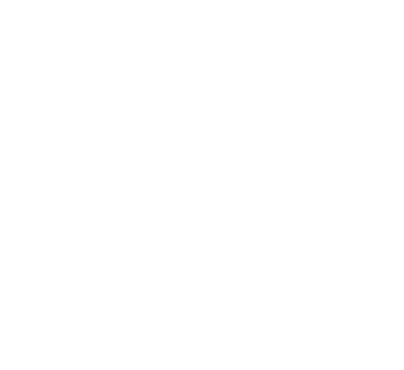1. AABC correctly drawn
2. Line y=x+1
BI
3. Triangle A'B'C'
Identifying coordinates of vertices of Δ A'B'C'
Δ A'B'C' correctly drawn
2.  Correct rotation of -90°
Correct vertices of Δ A"B"C"
Δ A'B'C' correctly drawn
3.
1. Oppositely Congruent
2. Directly Congruent

20.
1. AB =  10
8       20
AB = 1/2 x8
= 4 cm
2.
1. AC = √16+16 cm
=√32 cm
= 5.66 cm
2. height of pyramid
= √10² - (½ x 5.66)² = √(10² -2.83)²
= 9.59 cm

3. Volume of VABCD
1/3 x 4 x 4 x 4 x 9.59
= 51.15 cm³

Volume of VEFGH
= 1/3 x 8 x 8 x (2x9.59)
2x9.59 = 19.18
= 409.17

Volume of frustum ABCDEFGH
= 409.17–51.15
= 358.02cm2
21.
1. 2+8+10+6+2+x=40
x=12
2. Modal class = 180-189
3.
1. Mean
 Height Mid pt Freq fx 150-159160-169170-179180-189190-199200-209 154.5164.5174.5184.5194.5204.5 28101262 3091316174522141167409 40 7160

Mean height =
7160
40
= 179
2. Median
 U.C.B 159.5 169.5 179.5 189.5 199.5 209.5 C.F 2 10 20 32 38 40

Median Height = height of 20th athlete
Median = 1695 - ((40/20 - 10) /10) 10
= 179.5

22.
1. Let Z BDC = θ
Sin θ =  Sin 30°
5           4
Sin θ = 5 x Sin30 = 0.625
4
Acute θ = 38.68°
Obtuse θ = 141.32°
Angle ABD = 180-38.68x2
= 102.64
AD² = 4² + 4² - 2 x 4 x 4 cos 102.64
= 39

3. Length of DC
<DBC = 180-(30+141.32)
= 8.68°
Using sine rule
Sin 8.68 = Sin30
DC           4
DC = 8 Sin 8.68
= 1.21m
4. Area of ABC
= ½ x 4 x 5 Sin(8.68+102.64)
8.68° +102.64o=111 32
=9.32m2

23.
1.
 0 200 400 600 800 1000 1200 Ordinates along AB 200 240 280 300 280 240 200 Ordinates along CD 400 500 580 600 580* 580 640

2. Area of piece of land ABCD using trapezium rule
Area under curve AB
=1/2 x 200 {(200 + 200) + 2(240+280 +300 + 280 +240)}
For ordinates along CD Atx=800, accept 580 S y < 590
Use of differences
= 100 (400 + 2680)
= 308 000 m2
Area under curve CD
=½ - x200{(400 + 640) +2 (500 + 580 + 600 + 580 + 580)
= 100 (1040 + 5680)
=672 000
Area of land ABCD
= 672000 - 308000 = 364000 m
364000ha
10000
= 36.4 ha
3. Area using mid ordinate Rule:
= 400{(500+600 +580)-(240+300 + 240)}
= 400 x 900
= 360000 m2
= 360000
10000
= 36 ha

24.
1.
1. y = x3 + x2 - x - 1
dy/dx = 3x2 + 2x - 1
3x2 + 2x - 1 = 0 at stationary point.
(x + 1) (3x - 1) = 0
x = -1 Or 1/3
(-1,0) and (1/3, -15/27)

2. Nature of stationary points
d²y = 6x + 2
dx²
At x =-1
d²y = -6 + 2
dx²
=-4(Negative)
x= -1 is a maximum point.
d²y =  6  + 2
dx²     3
=4
At x= 1/3 is a minimum point
2.
1. At x = 1  y=0
at x=1
dy/dx = 3(1)+2(1) -1 = 4
Equation of tangent
y-0 = 4
x-1
y = 4x - 4

2. Let gradient of normal = m2
m2 x 4 = -1
m2 =  -1/4
y-0
x-1     4
y = -1/4x + 1/4